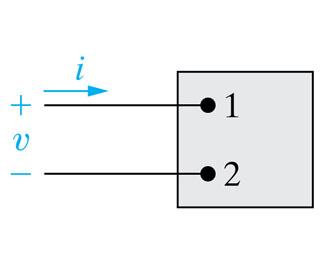Create an Account

Home / Questions / There is no charge at the upper terminal of the element in

There is no charge at the upper terminal of the element in

There is no charge at the upper terminal of the element in (Figure 1) for t < 0. At t = 0 a current of145e^(?2500t) mA , where t is in seconds, enters the upper terminal.^Figure 1^

A) Find the total charge that accumulates at the upper terminal.

qT= ?

B) If the current is stopped at t = 0.6ms , how much charge has accumulated at the upper terminal?

q= ?

Jul 31 2020 View more View LessSubscribe To Get Solution